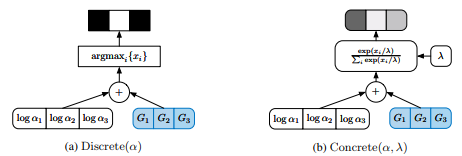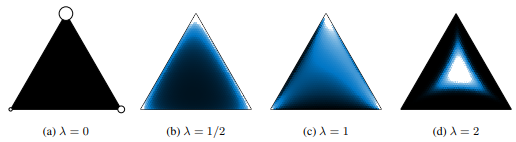# The Concrete Distribution: A Continuous Relaxation of Discrete Random Variables

## WHY?

Reparameterization trick is a useful technique for estimating gradient for loss function with stochastic variables. While score function extimators suffer from great variance, RT enable the gradient to be estimated with pathwise derivatives. Even though reparameterization trick can be applied to various kinds of random variables enabling backpropagation, it has not been applicable to discrete random variables.

## WHAT?

Reparameterization of discrete random variable is enabled by relaxing the condition of Categorical variables to Concrete variables(Continuous relaxations of discrete random variables). Concrete variable is motivated by Gumbel-Max trick. Gumbel distribution can be defined as -\log(-\log U), U \sim Uniform(0,1)$-\log(-\log U), U \sim Uniform(0,1)$. If we set D_k = 1$D_k = 1$ for such k that maximize {\log\alpha_k - \log(-\log U_k)}${\log\alpha_k - \log(-\log U_k)}$, then \mathbb{P}(D_k = 1) = \frac{\alpha_k}{\Sigma_{i=1}^n \alpha_i}$\mathbb{P}(D_k = 1) = \frac{\alpha_k}{\Sigma_{i=1}^n \alpha_i}$. This transforms the sampling of discrete random variable to deterministic transformation of uniform random variable.However, argmax process of Gunbel-Max trick is not appropriate for backpropagation. Concrete distribution substitute the argmax process with softmax with temperatures. This approahes to argmax as \lambda \rightarrow 0$\lambda \rightarrow 0$. The probability distribution is as follows.
X_k = \frac{exp((\log\alpha_k + G_k)/\lambda)}{\Sigma_{i=1}^n exp((\log\alpha_k + G_k)/\lambda)}\\ p_{\alpha,\lambda}(x) = (n-1)!\lambda^{n-1}\Pi_{k=1}^n(\frac{\alpha_k x_k^{\lambda - 1}}{\Sigma_{i=1}^n \alpha_i x_i^{-\lambda}})$X_k = \frac{exp((\log\alpha_k + G_k)/\lambda)}{\Sigma_{i=1}^n exp((\log\alpha_k + G_k)/\lambda)}\\ p_{\alpha,\lambda}(x) = (n-1)!\lambda^{n-1}\Pi_{k=1}^n(\frac{\alpha_k x_k^{\lambda - 1}}{\Sigma_{i=1}^n \alpha_i x_i^{-\lambda}})$We can use this Concrete random varibale in computing gradient of discrete stochastic variables. By substituting discete random variables with concrete random variables, variational lowerbound can be relaxed as follows. L_1(\theta, a, \alpha) = \mathbb{E}_{D\sim Q_{\alpha}(d|x)}[log\frac{p_\theta(x|D)P_a(D)}{Q_{\alpha}(D|x)}]\\ L_1(\theta, a, \alpha) \approx \mathbb{E}_{Z\sim q_{\alpha, \lambda_1}(z|x)}[log\frac{p_\theta(x|Z)p_{a,\lambda_2}(Z)}{q_{\alpha, \lambda_1}(Z|x)}]$L_1(\theta, a, \alpha) = \mathbb{E}_{D\sim Q_{\alpha}(d|x)}[log\frac{p_\theta(x|D)P_a(D)}{Q_{\alpha}(D|x)}]\\ L_1(\theta, a, \alpha) \approx \mathbb{E}_{Z\sim q_{\alpha, \lambda_1}(z|x)}[log\frac{p_\theta(x|Z)p_{a,\lambda_2}(Z)}{q_{\alpha, \lambda_1}(Z|x)}]$

## So?

Concrete relaxation outperformed VIMCO in structured output prediction and density estimation with non-linear model.

## Critic

Backpropagation of discrete stochastic random variables can be useful in other areas too. Maybe more various kinds of experiments would be nice.

Powered by aiden